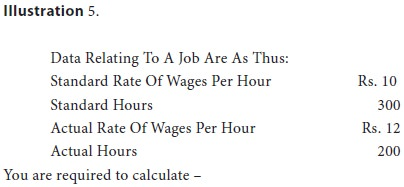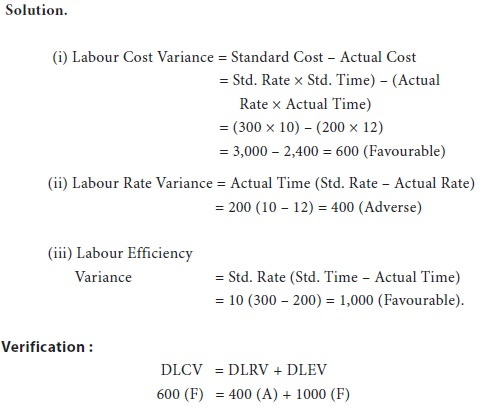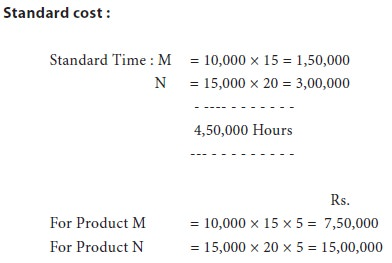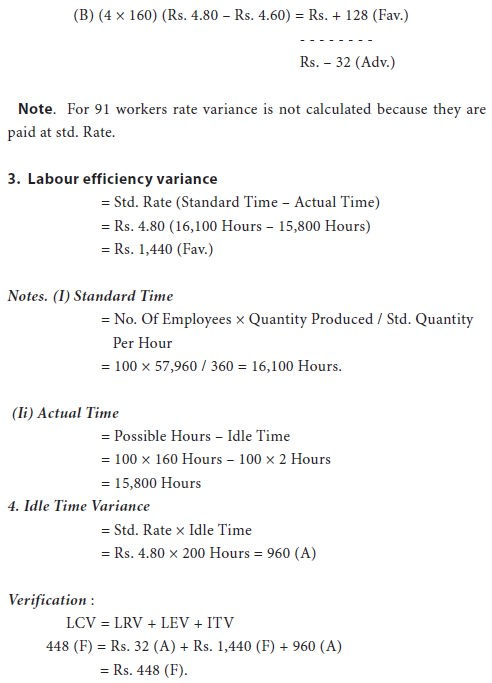Home | ARTS | Accounting For Managers | Direct Labour Cost Variance (DLCV)

# Direct Labour Cost Variance (DLCV)

Posted On :  04.05.2018 12:27 am

Labour variances are calculated like material variances. The direct labour cost variance is the difference between the standard direct wages specified for the activity achieved and the actual direct wages paid. The formula is :

Direct Labour Cost Variance (DLCV)

Labour variances are calculated like material variances. The direct labour cost variance is the difference between the standard direct wages specified for the activity achieved and the actual direct wages paid. The formula is :

DLCV = Standard Cost For Actual Output – Actual Cost or
=  (Standard Rate × Standard Time For Actual Output) – (Actual Rate × Actual Time)
=  (SR × ST) – (AR × AT)

Example 4 :

Standard Hours                                                    5,000

Standard Wage Rate                                   Rs.4 Per Unit

Actual Hours                                                       6,000

Actual Wage Rate                                        Rs.3.50 Per Unit

calculate labour cost variance.
Solution :

DLCV = (SR × ST) – (AR × AT)

=   (4 × 5,000) – (3.50 × 6,000)

=   20,000 – 21,000

The labour cost variance may arise on account of difference in either rates of wage or time. Thus, it may be analysed further as (i) labour rate variance, and (ii) labour time or efficiency variance.

## Direct Labour Rate Variance (DLRV)

It is the difference between the standard rate specified and the actual rate paid. It is also called ‘rate of pay variance or wage rate variance’. This would arise, usually, because of : (i) excessive overtime, (ii) employment of wrong type of labour (employing skilled person in place of an unskilled one), (iii) overtime workers engaged more or less than the standard, (iv) employment of labour at higher rates due to shortage of workers etc. The formula for calculating labour rate variance is as under :

Direct Labour Rate Variance = Actual Time (Standard Rate – Actual Rate)

DLRV = AT (SR – AR)

## Direct Labour Time Or Efficiency Variance (DLEV)

It is the difference between the standard labour hours specified and the actual hours spent on the works. This variance is primarily concerned with the standard wage rate. As such, where piece wage payment is in force, there will be no labour efficiency variance. Labour efficiency variance arises on account of any one or combination of factors such as : (i) lack of supervision, (ii) poor working conditions in the factory, (iii) use of sub-standard or higher standard materials, (iv) inefficiency of workers due to inadequate training, (v) lack of proper tools, equipment and machinery, (vi) higher labour turnover etc. Symbolically,

Labour Time Or Efficiency Variance = Standard Rate (Standard Time For Actual Output – Actual Time)
DLEV = SR (ST – AT)(i)   labour cost variance, (ii) labour rate variance and (iii) labour efficiency variance.Illustration 6.

Standard hours for manufacturing two products m and n are 15 hours per unit and 20 hours per unit respectively. Both products require identical kind of labour and the standard wage rate per hour is rs. 5. In the year 2011, 10,000 units of m and 15,000 units of n were manufactured. The total of labour hours actually worked were 4,50,500 and the actual wage bill came to rs. 23,00,000. This included 12,000 hours paid for @ rs. 7 per hour and 9,400 hours paid for @ rs. 7.50 per hour, the balance having been paid at rs. 5 per hour. You are required to compute the labour variances.

Solution :

LabourCostVariance=StandardCostForActualOutput–ActualCost## Idle Time Variance (ITV)

Idle time variance, a component of labour efficiency variance, is represented by the standard cost of the actual hours for which the workers remain idle due to abnormal circumstances, like non-availability of raw materials, power cut, breakdown of machinery etc. Symbolically :

Idle Time Variance = Standard Hourly Rate × Idle Time Or Hours
= SR × IT
This variance is always adverse. The total of labour rate, idle time and efficiency variances would be equal to labour cost variance, as shown below:

Illustration 7.

100 workers are working in a factory at a standard wage of rs. 4.80 per hour. During a month there are four weeks of 40 hours each. The standard performance is set at 360 units per hour. The following is the summary of the wages paid during the month:
91 workers were paid @ rs. 4.80 per hour

5 workers were paid @ rs. 5.00 per hour

The remaining were paid @ rs. 4.60 per hour

Power failure stopped production for 2 hours actual production 57,960 units. Calculate labour variances.

Solution.Tags : Accounting For Managers - Cost Estimation And Control-Standard Costing And Variance Analysis
Last 30 days 1485 views